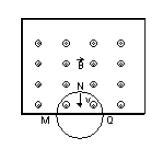#### A thin circular-conducting ring having N turns of radius R is falling with its plane vertical in a horizontal magnetic field B. At the position MNQ, the speed of ring is v, the induced e.m.f. developed across the ring isOption 1) Zero Option 2)and M is at higher potential Option 3)and Q is at higher potential Option 4)and Q is at lower potential

As we discussed in concept

Motional EMF -- whereinmagnetic fieldlengthvelocity of u perpendicular to uniform magnetic field.

Emf induced = (B.(MQ) v) N

= B . (2R) v N,

Q will be at lower potential.

Option 1)

Zero

This option is incorrect.

Option 2)and M is at higher potential

This option is incorrect.

Option 3)and Q is at higher potential

This option is incorrect.

Option 4)and Q is at lower potential

This option is correct.